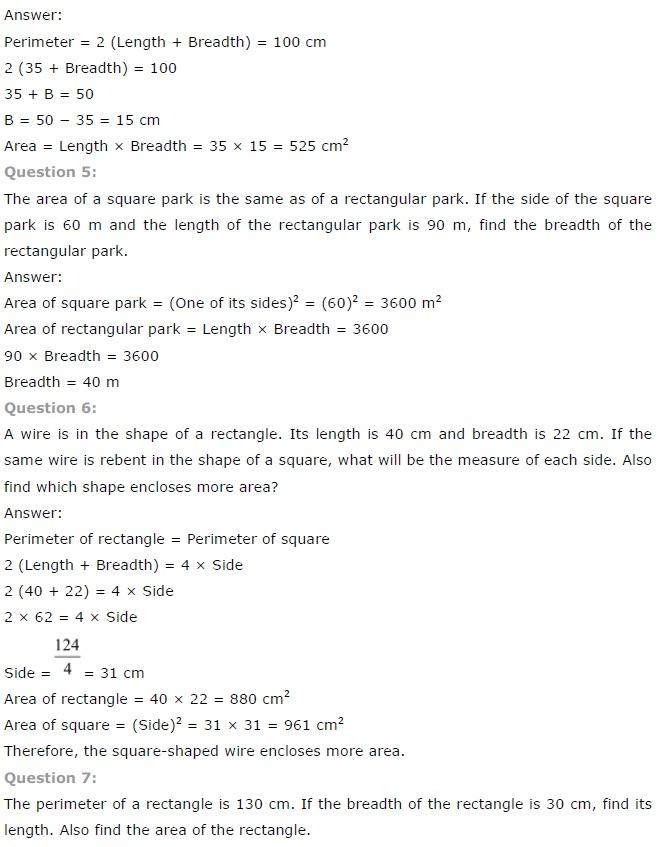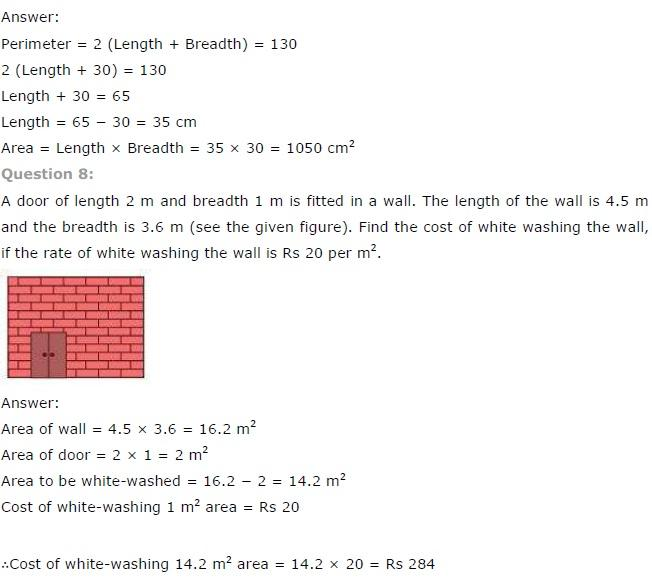# NCERT Solutions class 7 Maths Chapter-11 Exercise 11.1

## NCERT Solutions class 7 Maths Chapter-11 Perimeter and Area

### Perimeter and Area Exercise-11.1

NCERT Solutions Class-7 Maths chapter-11 Perimeter and Area Exercise-11.1 is prepared by academic team of pw all the questions of NCERT text book are solved step by step with proper and detail solutions explaining each and every questions . For More and additional questions of CBSE class 7 maths you can go to class 7 maths sections. NCERT class 7 Maths Solutions is the best way to enhanced your mathematics skill. And pw practice worksheet & question bank will help you a lot .

### Solutions of Chapter Perimeter and Area Exercise-11.1

Question 1:
The length and the breadth of a rectangular piece of land is 500 m by 300 m respectively.
Find:
(i) Its area
(ii) The cost of the land, if 1 m2 of the land costs Rs. 10,000.

It is given in the question that,
Length of rectangular piece of land = 500 m
Breadth of rectangular piece of land = 300 m
(i) We know that,
Area of rectangle = Length × Breadth
= 500 × 300
= 150000 m2
∴ Area of rectangular piece of land = 150000 m2
(ii) It is given in the question that,
Cost of 1 m2 land = Rs 10,000
Hence,
Cost of 150000 m2 land = 10000 × 150000
= Rs 1500000000

Question 2:
Find the area of a square park whose perimeter is 320 m.

It is given in the question that,
Perimeter of square park = 320 m
We know that,
Perimeter of square park = 4 × Length of the side of park
320 = 4 × Length of the side of the park
∴ Length of side of park = 320/4
= 80 m
We also know that,
Area of square = (Side)2
= (80)2
= 6400 m2

Question 3:
Find the breadth of a rectangular plot of land. If its area is 440 m2 and the length is 22 m.
Also find its perimeter.

It is given in the question that,
Length of the rectangular plot of land = 22 m
Area of rectangular plot of land = 440 m2
We know that,
Area of rectangle = Length × Breadth
=440/22
= 20 m
Breadth of the rectangular plot of land = 20 m
We know that:
Perimeter of rectangle = 2 (length + Breadth)
= 2 (22 + 20)
= 2 (42)
= 84 m
Hence, perimeter of rectangle = 84 m

Question 4:
The perimeter of a rectangular sheet is 100 cm. If the length is 35 cm, find its breadth. Also find the area.NCERT CLASS 7 MATHEMATICS SOLUTIONS